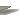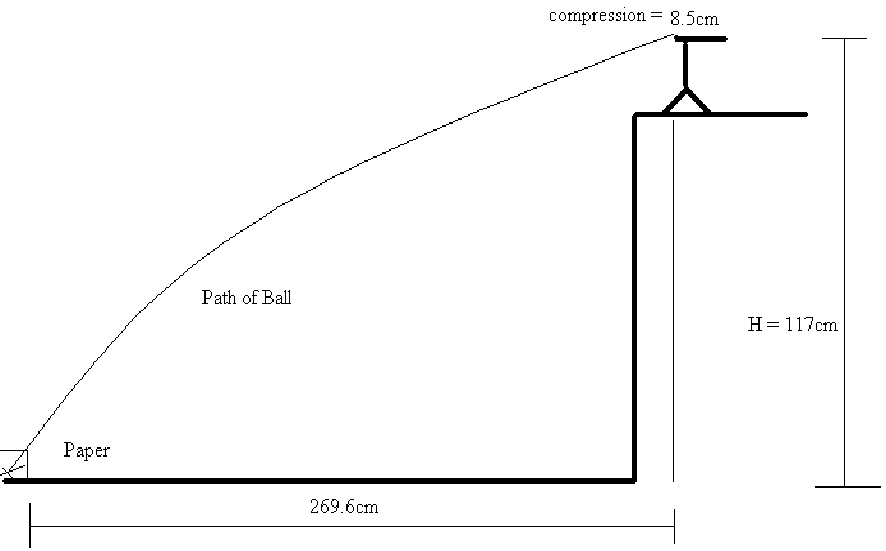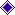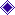Engineering Physics

Lab Report #3

## PH - 261 - 11

Projectile Motion 1

Andy Buettner

Mr. HuttlinObjective

The objective of this lab was to study the behavior of projectile motion using a spring launcher to launch a small mass from a certain height and recording the distance that it traveled.Materials

1. Spring launcher

2. Ball

3. Paper

4. Measuring apparatus

1. Meter stick

2. Tape Measure

5. Ink paperProcedure

1. # Set up apparatus

2. Measure total height of launcher

3. Use trials to estimate where the projectile will land

4. Place paper covered with an ink sheet to record where the projectile landed

5. Launch the Projectile

6. Repeat step 5 five times

7) Measure the individual distances of each trialData

1. Table 1:

 Trail # 1 2 3 4 5 Distance 282.8m 288.8m 290.3 291.6 286.6

II) Diagram 1:AnalysisNote: All calculations done on attached sheets1. Ravg = 288.1cm

2. V0 = 5.89 m / s

3. ak = 34.5 m / s2Conclusions

From this lab it was revealed that under the same circumstances, the same motion would occur and, by using basic Newtonian methods and mathematics, this motion could be predicted.Answers to Lab Questions

1. Q: Why can we reasonably assume that air resistance is negligible for this experiment?

A: We can be assured that air resistance is relatively negligible because the ball was mostly aerodynamic, and the velocities were relatively low.

1. Q: What effect would the air resistance have on the flight path if it were significant?

A: If the air resistance were significant, the ball wouldn't travel as far nor would it hit the ground as hardly. Also, the ball may not impact the paper at all because it could be pushed off course.

3) Q: Show that aavg = V02 / (2 * L)

A: Answer worked on attached sheet.